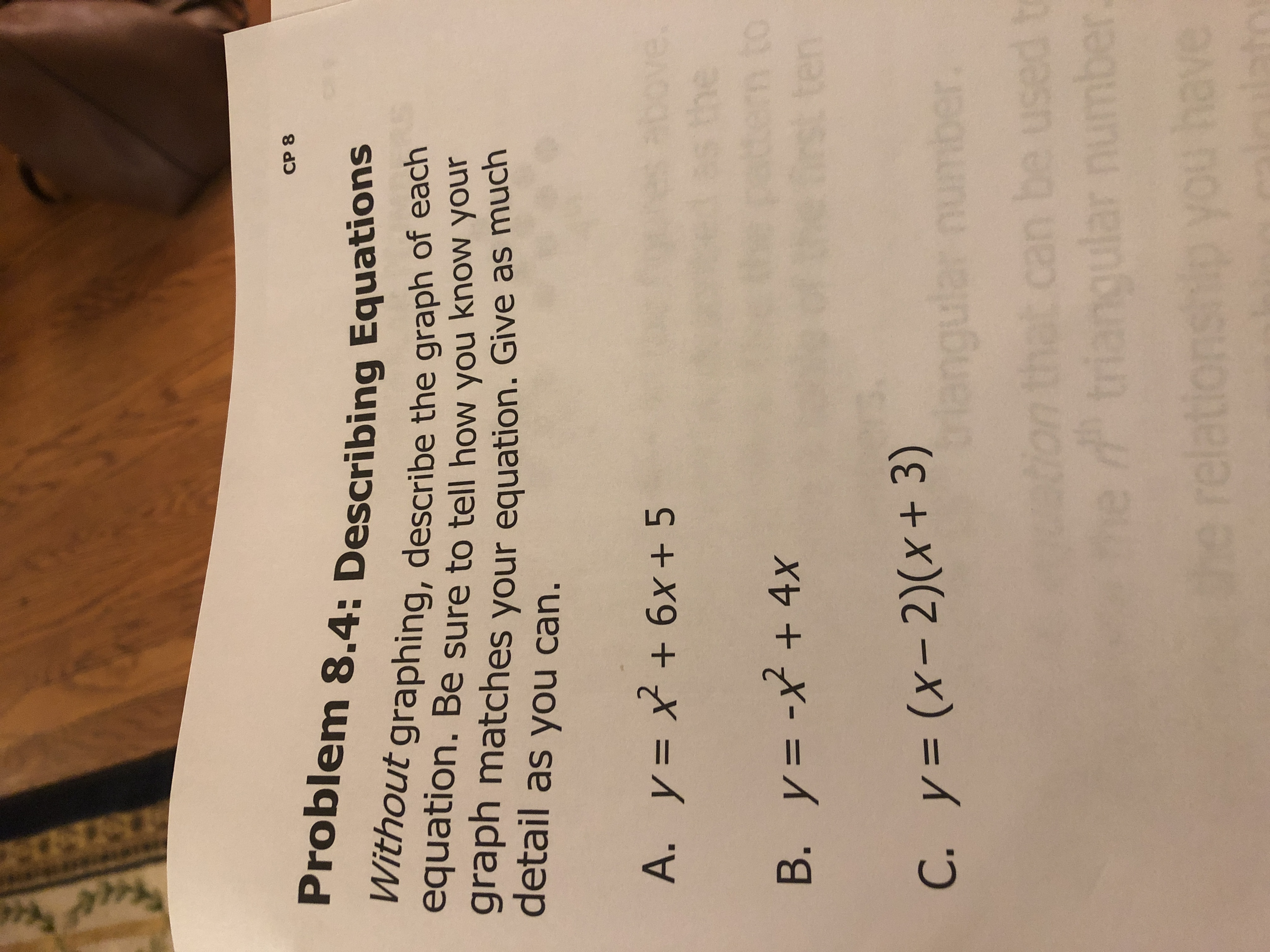# СР 8 Problem 8.4: Describing Equations Without graphing, describe the graph of each equation. Be sure to tell how you know your graph matches equation. Give as much detail as you can. your bove. the tem to ten A. y 6x+ 5 А. у - B. y= -x+ 4x C. y= (x- 2)(x + 3) g С. у%3D ton that can be used triangular number e ave Iotat relation

Questionhelp_outlineImage TranscriptioncloseСР 8 Problem 8.4: Describing Equations Without graphing, describe the graph of each equation. Be sure to tell how you know your graph matches equation. Give as much detail as you can. your bove. the tem to ten A. y 6x+ 5 А. у - B. y= -x+ 4x C. y= (x- 2)(x + 3) g С. у%3D ton that can be used triangular number e ave Iotat relation fullscreen

### Want to see this answer and more?

Experts are waiting 24/7 to provide step-by-step solutions in as fast as 30 minutes!*